# Stochastic Control/Reinforcement Learning for Optimal Market Making

26 Dec 2019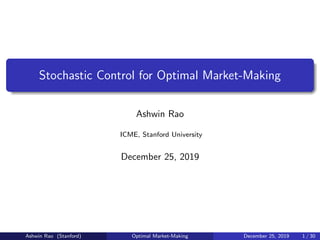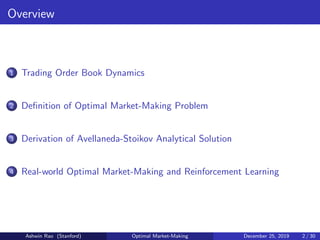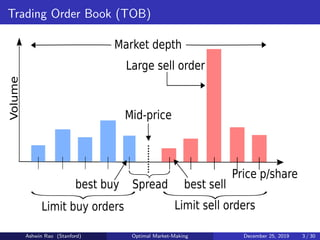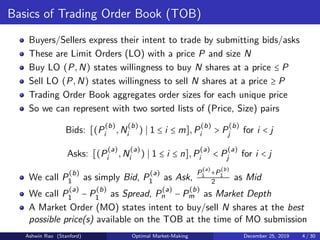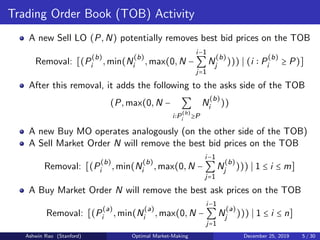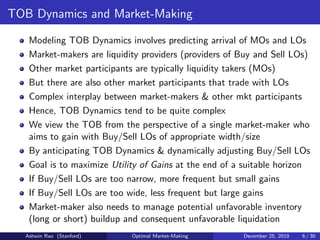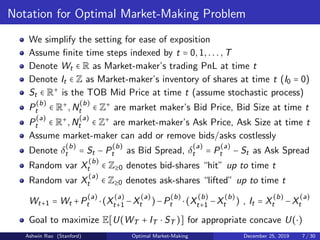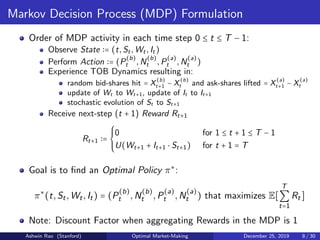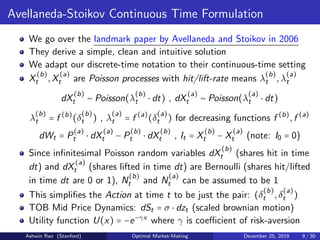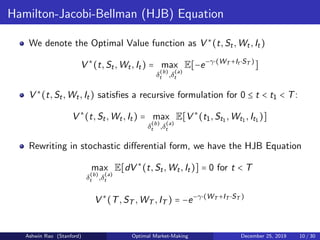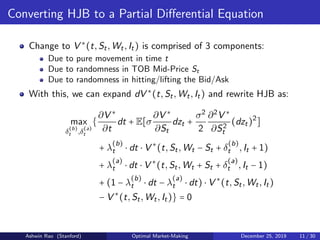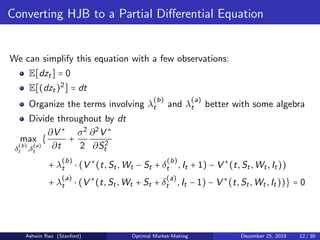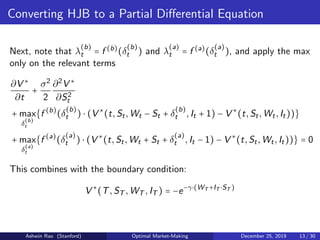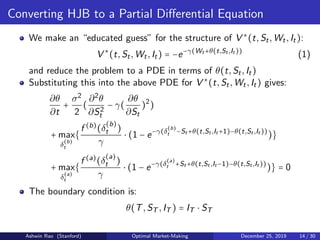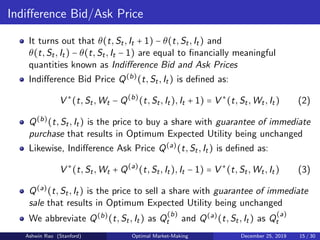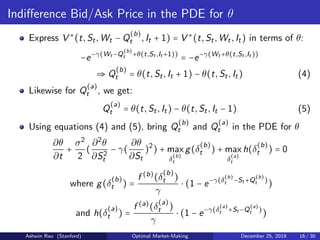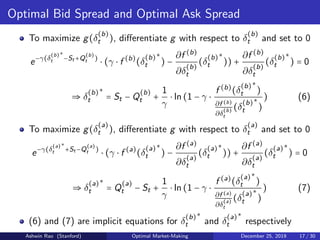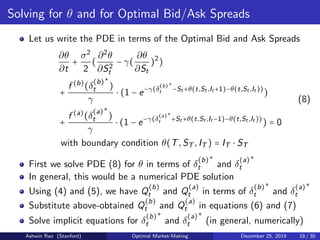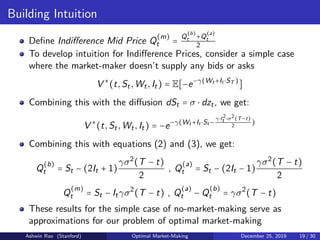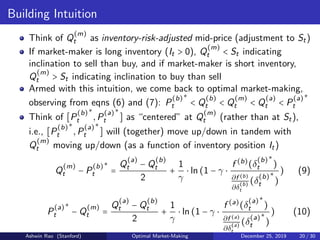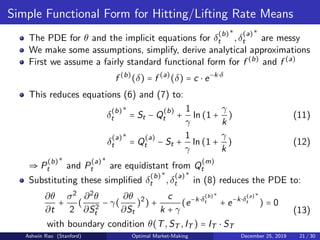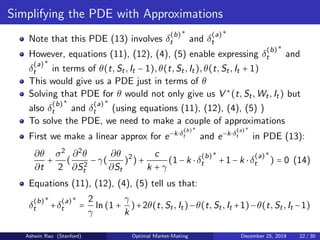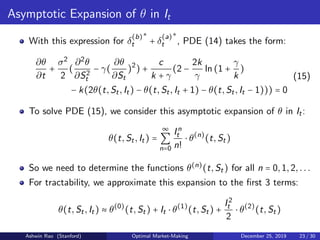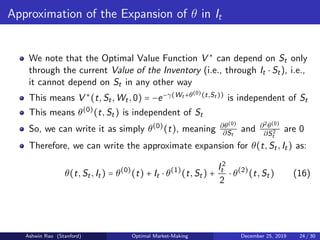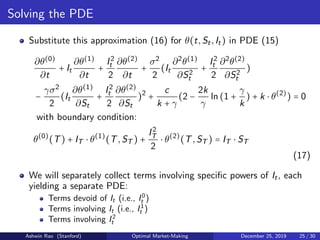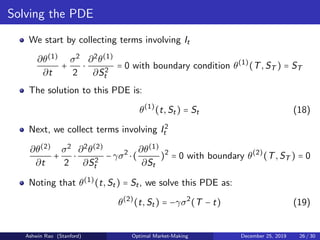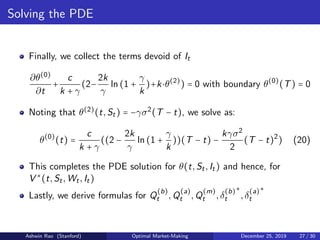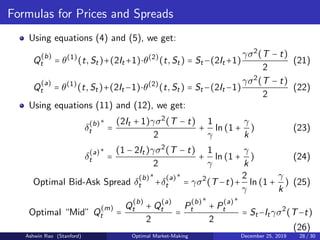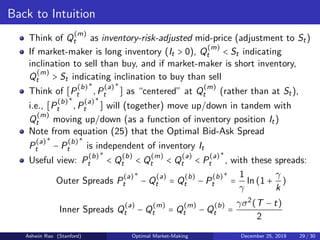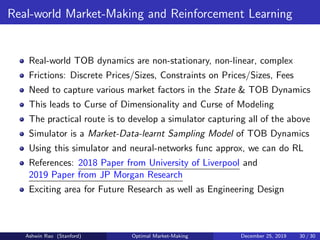1 sur 30

### Stochastic Control/Reinforcement Learning for Optimal Market Making

• 1. Stochastic Control for Optimal Market-Making Ashwin Rao ICME, Stanford University December 25, 2019 Ashwin Rao (Stanford) Optimal Market-Making December 25, 2019 1 / 30
• 2. Overview 1 Trading Order Book Dynamics 2 Deﬁnition of Optimal Market-Making Problem 3 Derivation of Avellaneda-Stoikov Analytical Solution 4 Real-world Optimal Market-Making and Reinforcement Learning Ashwin Rao (Stanford) Optimal Market-Making December 25, 2019 2 / 30
• 3. Trading Order Book (TOB) Ashwin Rao (Stanford) Optimal Market-Making December 25, 2019 3 / 30
• 5. Trading Order Book (TOB) Activity A new Sell LO (P,N) potentially removes best bid prices on the TOB Removal: [(P (b) i ,min(N (b) i ,max(0,N − i−1 ∑ j=1 N (b) j ))) (i P (b) i ≥ P)] After this removal, it adds the following to the asks side of the TOB (P,max(0,N − ∑ i P (b) i ≥P N (b) i )) A new Buy MO operates analogously (on the other side of the TOB) A Sell Market Order N will remove the best bid prices on the TOB Removal: [(P (b) i ,min(N (b) i ,max(0,N − i−1 ∑ j=1 N (b) j ))) 1 ≤ i ≤ m] A Buy Market Order N will remove the best ask prices on the TOB Removal: [(P (a) i ,min(N (a) i ,max(0,N − i−1 ∑ j=1 N (a) j ))) 1 ≤ i ≤ n] Ashwin Rao (Stanford) Optimal Market-Making December 25, 2019 5 / 30
• 6. TOB Dynamics and Market-Making Modeling TOB Dynamics involves predicting arrival of MOs and LOs Market-makers are liquidity providers (providers of Buy and Sell LOs) Other market participants are typically liquidity takers (MOs) But there are also other market participants that trade with LOs Complex interplay between market-makers & other mkt participants Hence, TOB Dynamics tend to be quite complex We view the TOB from the perspective of a single market-maker who aims to gain with Buy/Sell LOs of appropriate width/size By anticipating TOB Dynamics & dynamically adjusting Buy/Sell LOs Goal is to maximize Utility of Gains at the end of a suitable horizon If Buy/Sell LOs are too narrow, more frequent but small gains If Buy/Sell LOs are too wide, less frequent but large gains Market-maker also needs to manage potential unfavorable inventory (long or short) buildup and consequent unfavorable liquidation Ashwin Rao (Stanford) Optimal Market-Making December 25, 2019 6 / 30
• 7. Notation for Optimal Market-Making Problem We simplify the setting for ease of exposition Assume ﬁnite time steps indexed by t = 0,1,...,T Denote Wt ∈ R as Market-maker’s trading PnL at time t Denote It ∈ Z as Market-maker’s inventory of shares at time t (I0 = 0) St ∈ R+ is the TOB Mid Price at time t (assume stochastic process) P (b) t ∈ R+ ,N (b) t ∈ Z+ are market maker’s Bid Price, Bid Size at time t P (a) t ∈ R+ ,N (a) t ∈ Z+ are market-maker’s Ask Price, Ask Size at time t Assume market-maker can add or remove bids/asks costlessly Denote δ (b) t = St − P (b) t as Bid Spread, δ (a) t = P (a) t − St as Ask Spread Random var X (b) t ∈ Z≥0 denotes bid-shares “hit” up to time t Random var X (a) t ∈ Z≥0 denotes ask-shares “lifted” up to time t Wt+1 = Wt +P (a) t ⋅(X (a) t+1 −X (a) t )−P (b) t ⋅(X (b) t+1 −X (b) t ) , It = X (b) t −X (a) t Goal to maximize E[U(WT + IT ⋅ ST )] for appropriate concave U(⋅) Ashwin Rao (Stanford) Optimal Market-Making December 25, 2019 7 / 30
• 8. Markov Decision Process (MDP) Formulation Order of MDP activity in each time step 0 ≤ t ≤ T − 1: Observe State = (t,St,Wt,It) Perform Action = (P (b) t ,N (b) t ,P (a) t ,N (a) t ) Experience TOB Dynamics resulting in: random bid-shares hit = X (b) t+1 − X (b) t and ask-shares lifted = X (a) t+1 − X (a) t update of Wt to Wt+1, update of It to It+1 stochastic evolution of St to St+1 Receive next-step (t + 1) Reward Rt+1 Rt+1 = ⎧⎪⎪ ⎨ ⎪⎪⎩ 0 for 1 ≤ t + 1 ≤ T − 1 U(Wt+1 + It+1 ⋅ St+1) for t + 1 = T Goal is to ﬁnd an Optimal Policy π∗ : π∗ (t,St,Wt,It) = (P (b) t ,N (b) t ,P (a) t ,N (a) t ) that maximizes E[ T ∑ t=1 Rt] Note: Discount Factor when aggregating Rewards in the MDP is 1 Ashwin Rao (Stanford) Optimal Market-Making December 25, 2019 8 / 30
• 9. Avellaneda-Stoikov Continuous Time Formulation We go over the landmark paper by Avellaneda and Stoikov in 2006 They derive a simple, clean and intuitive solution We adapt our discrete-time notation to their continuous-time setting X (b) t ,X (a) t are Poisson processes with hit/lift-rate means λ (b) t ,λ (a) t dX (b) t ∼ Poisson(λ (b) t ⋅ dt) , dX (a) t ∼ Poisson(λ (a) t ⋅ dt) λ (b) t = f (b) (δ (b) t ) , λ (a) t = f (a) (δ (a) t ) for decreasing functions f (b) ,f (a) dWt = P (a) t ⋅ dX (a) t − P (b) t ⋅ dX (b) t , It = X (b) t − X (a) t (note: I0 = 0) Since inﬁnitesimal Poisson random variables dX (b) t (shares hit in time dt) and dX (a) t (shares lifted in time dt) are Bernoulli (shares hit/lifted in time dt are 0 or 1), N (b) t and N (a) t can be assumed to be 1 This simpliﬁes the Action at time t to be just the pair: (δ (b) t ,δ (a) t ) TOB Mid Price Dynamics: dSt = σ ⋅ dzt (scaled brownian motion) Utility function U(x) = −e−γx where γ is coeﬃcient of risk-aversion Ashwin Rao (Stanford) Optimal Market-Making December 25, 2019 9 / 30
• 10. Hamilton-Jacobi-Bellman (HJB) Equation We denote the Optimal Value function as V ∗ (t,St,Wt,It) V ∗ (t,St,Wt,It) = max δ (b) t ,δ (a) t E[−e−γ⋅(WT +It ⋅ST ) ] V ∗ (t,St,Wt,It) satisﬁes a recursive formulation for 0 ≤ t < t1 < T: V ∗ (t,St,Wt,It) = max δ (b) t ,δ (a) t E[V ∗ (t1,St1 ,Wt1 ,It1 )] Rewriting in stochastic diﬀerential form, we have the HJB Equation max δ (b) t ,δ (a) t E[dV ∗ (t,St,Wt,It)] = 0 for t < T V ∗ (T,ST ,WT ,IT ) = −e−γ⋅(WT +IT ⋅ST ) Ashwin Rao (Stanford) Optimal Market-Making December 25, 2019 10 / 30
• 11. Converting HJB to a Partial Diﬀerential Equation Change to V ∗ (t,St,Wt,It) is comprised of 3 components: Due to pure movement in time t Due to randomness in TOB Mid-Price St Due to randomness in hitting/lifting the Bid/Ask With this, we can expand dV ∗ (t,St,Wt,It) and rewrite HJB as: max δ (b) t ,δ (a) t { ∂V ∗ ∂t dt + E[σ ∂V ∗ ∂St dzt + σ2 2 ∂2 V ∗ ∂S2 t (dzt)2 ] + λ (b) t ⋅ dt ⋅ V ∗ (t,St,Wt − St + δ (b) t ,It + 1) + λ (a) t ⋅ dt ⋅ V ∗ (t,St,Wt + St + δ (a) t ,It − 1) + (1 − λ (b) t ⋅ dt − λ (a) t ⋅ dt) ⋅ V ∗ (t,St,Wt,It) − V ∗ (t,St,Wt,It)} = 0 Ashwin Rao (Stanford) Optimal Market-Making December 25, 2019 11 / 30
• 12. Converting HJB to a Partial Diﬀerential Equation We can simplify this equation with a few observations: E[dzt] = 0 E[(dzt)2 ] = dt Organize the terms involving λ (b) t and λ (a) t better with some algebra Divide throughout by dt max δ (b) t ,δ (a) t { ∂V ∗ ∂t + σ2 2 ∂2 V ∗ ∂S2 t + λ (b) t ⋅ (V ∗ (t,St,Wt − St + δ (b) t ,It + 1) − V ∗ (t,St,Wt,It)) + λ (a) t ⋅ (V ∗ (t,St,Wt + St + δ (a) t ,It − 1) − V ∗ (t,St,Wt,It))} = 0 Ashwin Rao (Stanford) Optimal Market-Making December 25, 2019 12 / 30
• 13. Converting HJB to a Partial Diﬀerential Equation Next, note that λ (b) t = f (b) (δ (b) t ) and λ (a) t = f (a) (δ (a) t ), and apply the max only on the relevant terms ∂V ∗ ∂t + σ2 2 ∂2 V ∗ ∂S2 t + max δ (b) t {f (b) (δ (b) t ) ⋅ (V ∗ (t,St,Wt − St + δ (b) t ,It + 1) − V ∗ (t,St,Wt,It))} + max δ (a) t {f (a) (δ (a) t ) ⋅ (V ∗ (t,St,Wt + St + δ (a) t ,It − 1) − V ∗ (t,St,Wt,It))} = 0 This combines with the boundary condition: V ∗ (T,ST ,WT ,IT ) = −e−γ⋅(WT +IT ⋅ST ) Ashwin Rao (Stanford) Optimal Market-Making December 25, 2019 13 / 30
• 14. Converting HJB to a Partial Diﬀerential Equation We make an “educated guess” for the structure of V ∗ (t,St,Wt,It): V ∗ (t,St,Wt,It) = −e−γ(Wt +θ(t,St ,It )) (1) and reduce the problem to a PDE in terms of θ(t,St,It) Substituting this into the above PDE for V ∗ (t,St,Wt,It) gives: ∂θ ∂t + σ2 2 ( ∂2 θ ∂S2 t − γ( ∂θ ∂St )2 ) + max δ (b) t { f (b) (δ (b) t ) γ ⋅ (1 − e−γ(δ (b) t −St +θ(t,St ,It +1)−θ(t,St ,It )) )} + max δ (a) t { f (a) (δ (a) t ) γ ⋅ (1 − e−γ(δ (a) t +St +θ(t,St ,It −1)−θ(t,St ,It )) )} = 0 The boundary condition is: θ(T,ST ,IT ) = IT ⋅ ST Ashwin Rao (Stanford) Optimal Market-Making December 25, 2019 14 / 30
• 15. Indiﬀerence Bid/Ask Price It turns out that θ(t,St,It + 1) − θ(t,St,It) and θ(t,St,It) − θ(t,St,It − 1) are equal to ﬁnancially meaningful quantities known as Indiﬀerence Bid and Ask Prices Indiﬀerence Bid Price Q(b) (t,St,It) is deﬁned as: V ∗ (t,St,Wt − Q(b) (t,St,It),It + 1) = V ∗ (t,St,Wt,It) (2) Q(b) (t,St,It) is the price to buy a share with guarantee of immediate purchase that results in Optimum Expected Utility being unchanged Likewise, Indiﬀerence Ask Price Q(a) (t,St,It) is deﬁned as: V ∗ (t,St,Wt + Q(a) (t,St,It),It − 1) = V ∗ (t,St,Wt,It) (3) Q(a) (t,St,It) is the price to sell a share with guarantee of immediate sale that results in Optimum Expected Utility being unchanged We abbreviate Q(b) (t,St,It) as Q (b) t and Q(a) (t,St,It) as Q (a) t Ashwin Rao (Stanford) Optimal Market-Making December 25, 2019 15 / 30
• 16. Indiﬀerence Bid/Ask Price in the PDE for θ Express V ∗ (t,St,Wt − Q (b) t ,It + 1) = V ∗ (t,St,Wt,It) in terms of θ: −e−γ(Wt −Q (b) t +θ(t,St ,It +1)) = −e−γ(Wt +θ(t,St ,It )) ⇒ Q (b) t = θ(t,St,It + 1) − θ(t,St,It) (4) Likewise for Q (a) t , we get: Q (a) t = θ(t,St,It) − θ(t,St,It − 1) (5) Using equations (4) and (5), bring Q (b) t and Q (a) t in the PDE for θ ∂θ ∂t + σ2 2 ( ∂2 θ ∂S2 t − γ( ∂θ ∂St )2 ) + max δ (b) t g(δ (b) t ) + max δ (a) t h(δ (b) t ) = 0 where g(δ (b) t ) = f (b) (δ (b) t ) γ ⋅ (1 − e−γ(δ (b) t −St +Q (b) t ) ) and h(δ (a) t ) = f (a) (δ (a) t ) γ ⋅ (1 − e−γ(δ (a) t +St −Q (a) t ) ) Ashwin Rao (Stanford) Optimal Market-Making December 25, 2019 16 / 30
• 17. Optimal Bid Spread and Optimal Ask Spread To maximize g(δ (b) t ), diﬀerentiate g with respect to δ (b) t and set to 0 e−γ(δ (b) t ∗ −St +Q (b) t ) ⋅ (γ ⋅ f (b) (δ (b) t ∗ ) − ∂f (b) ∂δ (b) t (δ (b) t ∗ )) + ∂f (b) ∂δ (b) t (δ (b) t ∗ ) = 0 ⇒ δ (b) t ∗ = St − Q (b) t + 1 γ ⋅ ln(1 − γ ⋅ f (b) (δ (b) t ∗ ) ∂f (b) ∂δ (b) t (δ (b) t ∗ ) ) (6) To maximize g(δ (a) t ), diﬀerentiate g with respect to δ (a) t and set to 0 e−γ(δ (a) t ∗ +St −Q (a) t ) ⋅ (γ ⋅ f (a) (δ (a) t ∗ ) − ∂f (a) ∂δ (a) t (δ (a) t ∗ )) + ∂f (a) ∂δ (a) t (δ (a) t ∗ ) = 0 ⇒ δ (a) t ∗ = Q (a) t − St + 1 γ ⋅ ln(1 − γ ⋅ f (a) (δ (a) t ∗ ) ∂f (a) ∂δ (a) t (δ (a) t ∗ ) ) (7) (6) and (7) are implicit equations for δ (b) t ∗ and δ (a) t ∗ respectively Ashwin Rao (Stanford) Optimal Market-Making December 25, 2019 17 / 30
• 18. Solving for θ and for Optimal Bid/Ask Spreads Let us write the PDE in terms of the Optimal Bid and Ask Spreads ∂θ ∂t + σ2 2 ( ∂2 θ ∂S2 t − γ( ∂θ ∂St )2 ) + f (b) (δ (b) t ∗ ) γ ⋅ (1 − e−γ(δ (b) t ∗ −St +θ(t,St ,It +1)−θ(t,St ,It )) ) + f (a) (δ (a) t ∗ ) γ ⋅ (1 − e−γ(δ (a) t ∗ +St +θ(t,St ,It −1)−θ(t,St ,It )) ) = 0 with boundary condition θ(T,ST ,IT ) = IT ⋅ ST (8) First we solve PDE (8) for θ in terms of δ (b) t ∗ and δ (a) t ∗ In general, this would be a numerical PDE solution Using (4) and (5), we have Q (b) t and Q (a) t in terms of δ (b) t ∗ and δ (a) t ∗ Substitute above-obtained Q (b) t and Q (a) t in equations (6) and (7) Solve implicit equations for δ (b) t ∗ and δ (a) t ∗ (in general, numerically) Ashwin Rao (Stanford) Optimal Market-Making December 25, 2019 18 / 30
• 19. Building Intuition Deﬁne Indiﬀerence Mid Price Q (m) t = Q (b) t +Q (a) t 2 To develop intuition for Indiﬀerence Prices, consider a simple case where the market-maker doesn’t supply any bids or asks V ∗ (t,St,Wt,It) = E[−e−γ(Wt +It ⋅ST ) ] Combining this with the diﬀusion dSt = σ ⋅ dzt, we get: V ∗ (t,St,Wt,It) = −e−γ(Wt +It ⋅St − γ⋅I2 t ⋅σ2(T−t) 2 ) Combining this with equations (2) and (3), we get: Q (b) t = St − (2It + 1) γσ2 (T − t) 2 , Q (a) t = St − (2It − 1) γσ2 (T − t) 2 Q (m) t = St − Itγσ2 (T − t) , Q (a) t − Q (b) t = γσ2 (T − t) These results for the simple case of no-market-making serve as approximations for our problem of optimal market-making Ashwin Rao (Stanford) Optimal Market-Making December 25, 2019 19 / 30
• 20. Building Intuition Think of Q (m) t as inventory-risk-adjusted mid-price (adjustment to St) If market-maker is long inventory (It > 0), Q (m) t < St indicating inclination to sell than buy, and if market-maker is short inventory, Q (m) t > St indicating inclination to buy than sell Armed with this intuition, we come back to optimal market-making, observing from eqns (6) and (7): P (b) t ∗ < Q (b) t < Q (m) t < Q (a) t < P (a) t ∗ Think of [P (b) t ∗ ,P (a) t ∗ ] as “centered” at Q (m) t (rather than at St), i.e., [P (b) t ∗ ,P (a) t ∗ ] will (together) move up/down in tandem with Q (m) t moving up/down (as a function of inventory position It) Q (m) t − P (b) t ∗ = Q (a) t − Q (b) t 2 + 1 γ ⋅ ln(1 − γ ⋅ f (b) (δ (b) t ∗ ) ∂f (b) ∂δ (b) t (δ (b) t ∗ ) ) (9) P (a) t ∗ − Q (m) t = Q (a) t − Q (b) t 2 + 1 γ ⋅ ln(1 − γ ⋅ f (a) (δ (a) t ∗ ) ∂f (a) ∂δ (a) t (δ (a) t ∗ ) ) (10) Ashwin Rao (Stanford) Optimal Market-Making December 25, 2019 20 / 30
• 21. Simple Functional Form for Hitting/Lifting Rate Means The PDE for θ and the implicit equations for δ (b) t ∗ ,δ (a) t ∗ are messy We make some assumptions, simplify, derive analytical approximations First we assume a fairly standard functional form for f (b) and f (a) f (b) (δ) = f (a) (δ) = c ⋅ e−k⋅δ This reduces equations (6) and (7) to: δ (b) t ∗ = St − Q (b) t + 1 γ ln(1 + γ k ) (11) δ (a) t ∗ = Q (a) t − St + 1 γ ln(1 + γ k ) (12) ⇒ P (b) t ∗ and P (a) t ∗ are equidistant from Q (m) t Substituting these simpliﬁed δ (b) t ∗ ,δ (a) t ∗ in (8) reduces the PDE to: ∂θ ∂t + σ2 2 ( ∂2 θ ∂S2 t − γ( ∂θ ∂St )2 ) + c k + γ (e−k⋅δ (b) t ∗ + e−k⋅δ (a) t ∗ ) = 0 with boundary condition θ(T,ST ,IT ) = IT ⋅ ST (13) Ashwin Rao (Stanford) Optimal Market-Making December 25, 2019 21 / 30
• 22. Simplifying the PDE with Approximations Note that this PDE (13) involves δ (b) t ∗ and δ (a) t ∗ However, equations (11), (12), (4), (5) enable expressing δ (b) t ∗ and δ (a) t ∗ in terms of θ(t,St,It − 1),θ(t,St,It),θ(t,St,It + 1) This would give us a PDE just in terms of θ Solving that PDE for θ would not only give us V ∗ (t,St,Wt,It) but also δ (b) t ∗ and δ (a) t ∗ (using equations (11), (12), (4), (5) ) To solve the PDE, we need to make a couple of approximations First we make a linear approx for e−k⋅δ (b) t ∗ and e−k⋅δ (a) t ∗ in PDE (13): ∂θ ∂t + σ2 2 ( ∂2 θ ∂S2 t − γ( ∂θ ∂St )2 ) + c k + γ (1 − k ⋅ δ (b) t ∗ + 1 − k ⋅ δ (a) t ∗ ) = 0 (14) Equations (11), (12), (4), (5) tell us that: δ (b) t ∗ +δ (a) t ∗ = 2 γ ln(1 + γ k )+2θ(t,St,It)−θ(t,St,It +1)−θ(t,St,It −1) Ashwin Rao (Stanford) Optimal Market-Making December 25, 2019 22 / 30
• 23. Asymptotic Expansion of θ in It With this expression for δ (b) t ∗ + δ (a) t ∗ , PDE (14) takes the form: ∂θ ∂t + σ2 2 ( ∂2 θ ∂S2 t − γ( ∂θ ∂St )2 ) + c k + γ (2 − 2k γ ln(1 + γ k ) − k(2θ(t,St,It) − θ(t,St,It + 1) − θ(t,St,It − 1))) = 0 (15) To solve PDE (15), we consider this asymptotic expansion of θ in It: θ(t,St,It) = ∞ ∑ n=0 In t n! ⋅ θ(n) (t,St) So we need to determine the functions θ(n) (t,St) for all n = 0,1,2,... For tractability, we approximate this expansion to the ﬁrst 3 terms: θ(t,St,It) ≈ θ(0) (t,St) + It ⋅ θ(1) (t,St) + I2 t 2 ⋅ θ(2) (t,St) Ashwin Rao (Stanford) Optimal Market-Making December 25, 2019 23 / 30
• 24. Approximation of the Expansion of θ in It We note that the Optimal Value Function V ∗ can depend on St only through the current Value of the Inventory (i.e., through It ⋅ St), i.e., it cannot depend on St in any other way This means V ∗ (t,St,Wt,0) = −e−γ(Wt +θ(0)(t,St )) is independent of St This means θ(0) (t,St) is independent of St So, we can write it as simply θ(0) (t), meaning ∂θ(0) ∂St and ∂2θ(0) ∂S2 t are 0 Therefore, we can write the approximate expansion for θ(t,St,It) as: θ(t,St,It) = θ(0) (t) + It ⋅ θ(1) (t,St) + I2 t 2 ⋅ θ(2) (t,St) (16) Ashwin Rao (Stanford) Optimal Market-Making December 25, 2019 24 / 30
• 25. Solving the PDE Substitute this approximation (16) for θ(t,St,It) in PDE (15) ∂θ(0) ∂t + It ∂θ(1) ∂t + I2 t 2 ∂θ(2) ∂t + σ2 2 (It ∂2 θ(1) ∂S2 t + I2 t 2 ∂2 θ(2) ∂S2 t ) − γσ2 2 (It ∂θ(1) ∂St + I2 t 2 ∂θ(2) ∂St )2 + c k + γ (2 − 2k γ ln(1 + γ k ) + k ⋅ θ(2) ) = 0 with boundary condition: θ(0) (T) + IT ⋅ θ(1) (T,ST ) + I2 T 2 ⋅ θ(2) (T,ST ) = IT ⋅ ST (17) We will separately collect terms involving speciﬁc powers of It, each yielding a separate PDE: Terms devoid of It (i.e., I0 t ) Terms involving It (i.e., I1 t ) Terms involving I2 t Ashwin Rao (Stanford) Optimal Market-Making December 25, 2019 25 / 30
• 26. Solving the PDE We start by collecting terms involving It ∂θ(1) ∂t + σ2 2 ⋅ ∂2 θ(1) ∂S2 t = 0 with boundary condition θ(1) (T,ST ) = ST The solution to this PDE is: θ(1) (t,St) = St (18) Next, we collect terms involving I2 t ∂θ(2) ∂t + σ2 2 ⋅ ∂2 θ(2) ∂S2 t −γσ2 ⋅( ∂θ(1) ∂St )2 = 0 with boundary θ(2) (T,ST ) = 0 Noting that θ(1) (t,St) = St, we solve this PDE as: θ(2) (t,St) = −γσ2 (T − t) (19) Ashwin Rao (Stanford) Optimal Market-Making December 25, 2019 26 / 30
• 27. Solving the PDE Finally, we collect the terms devoid of It ∂θ(0) ∂t + c k + γ (2− 2k γ ln(1 + γ k )+k⋅θ(2) ) = 0 with boundary θ(0) (T) = 0 Noting that θ(2) (t,St) = −γσ2 (T − t), we solve as: θ(0) (t) = c k + γ ((2 − 2k γ ln(1 + γ k ))(T − t) − kγσ2 2 (T − t)2 ) (20) This completes the PDE solution for θ(t,St,It) and hence, for V ∗ (t,St,Wt,It) Lastly, we derive formulas for Q (b) t ,Q (a) t ,Q (m) t ,δ (b) t ∗ ,δ (a) t ∗ Ashwin Rao (Stanford) Optimal Market-Making December 25, 2019 27 / 30
• 28. Formulas for Prices and Spreads Using equations (4) and (5), we get: Q (b) t = θ(1) (t,St)+(2It +1)⋅θ(2) (t,St) = St −(2It +1) γσ2 (T − t) 2 (21) Q (a) t = θ(1) (t,St)+(2It −1)⋅θ(2) (t,St) = St −(2It −1) γσ2 (T − t) 2 (22) Using equations (11) and (12), we get: δ (b) t ∗ = (2It + 1)γσ2 (T − t) 2 + 1 γ ln(1 + γ k ) (23) δ (a) t ∗ = (1 − 2It)γσ2 (T − t) 2 + 1 γ ln(1 + γ k ) (24) Optimal Bid-Ask Spread δ (b) t ∗ +δ (a) t ∗ = γσ2 (T −t)+ 2 γ ln(1 + γ k ) (25) Optimal “Mid” Q (m) t = Q (b) t + Q (a) t 2 = P (b) t ∗ + P (a) t ∗ 2 = St−Itγσ2 (T−t) (26) Ashwin Rao (Stanford) Optimal Market-Making December 25, 2019 28 / 30
• 29. Back to Intuition Think of Q (m) t as inventory-risk-adjusted mid-price (adjustment to St) If market-maker is long inventory (It > 0), Q (m) t < St indicating inclination to sell than buy, and if market-maker is short inventory, Q (m) t > St indicating inclination to buy than sell Think of [P (b) t ∗ ,P (a) t ∗ ] as “centered” at Q (m) t (rather than at St), i.e., [P (b) t ∗ ,P (a) t ∗ ] will (together) move up/down in tandem with Q (m) t moving up/down (as a function of inventory position It) Note from equation (25) that the Optimal Bid-Ask Spread P (a) t ∗ − P (b) t ∗ is independent of inventory It Useful view: P (b) t ∗ < Q (b) t < Q (m) t < Q (a) t < P (a) t ∗ , with these spreads: Outer Spreads P (a) t ∗ − Q (a) t = Q (b) t − P (b) t ∗ = 1 γ ln(1 + γ k ) Inner Spreads Q (a) t − Q (m) t = Q (m) t − Q (b) t = γσ2 (T − t) 2 Ashwin Rao (Stanford) Optimal Market-Making December 25, 2019 29 / 30
• 30. Real-world Market-Making and Reinforcement Learning Real-world TOB dynamics are non-stationary, non-linear, complex Frictions: Discrete Prices/Sizes, Constraints on Prices/Sizes, Fees Need to capture various market factors in the State & TOB Dynamics This leads to Curse of Dimensionality and Curse of Modeling The practical route is to develop a simulator capturing all of the above Simulator is a Market-Data-learnt Sampling Model of TOB Dynamics Using this simulator and neural-networks func approx, we can do RL References: 2018 Paper from University of Liverpool and 2019 Paper from JP Morgan Research Exciting area for Future Research as well as Engineering Design Ashwin Rao (Stanford) Optimal Market-Making December 25, 2019 30 / 30